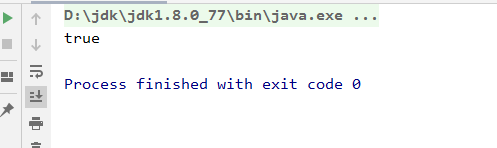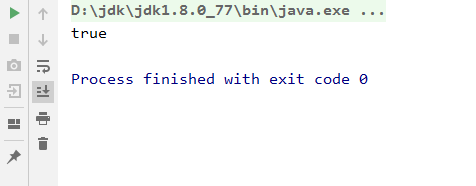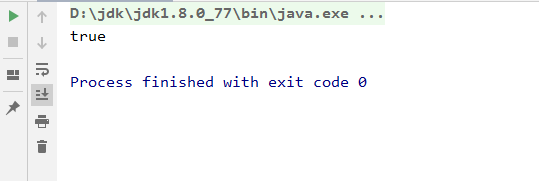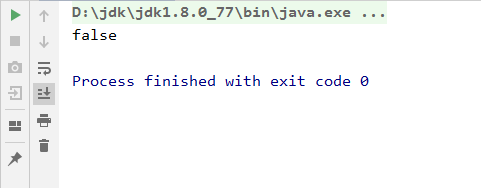# Java的值类型和引用类型Java的值类型和引用类型# 二、值类型和引用类型的比较

## 引用类型```1     /**
2      * 测试Integer
3      */
4     public static void test_Integer(){
5         Integer number_01 = 10;
6         Integer number_02 = 10;
7         System.out.println(number_01.equals(number_02));
8     }```## 值类型```1     /**
2      * 测试int
3      */
4     public static void test_Int(){
5         int number_01 = 10;
6         int number_02 = 10;
7         System.out.println(number_01 == number_02);
8     }```# 三、问题

## 初始没问题的情况```1     /**
2      * 测试Integer
3      */
4     public static void test_Integer(){
5         Integer number_001 = 10;
6         Integer number_002 = 10;
7         System.out.println(number_001 == number_002);
8     }```## 当记录超过一定数的时候，出现问题```1     /**
2      * 测试Integer
3      */
4     public static void test_Integer(){
5         Integer number_001 = 128;
6         Integer number_002 = 128;
7         System.out.println(number_001 == number_002);
8     }```# 四、解决``` 1 private static class IntegerCache {
2         static final int low = -128;
3         static final int high;
4         static final Integer cache[];
5
6         static {
7             // high value may be configured by property
8             int h = 127;
9             String integerCacheHighPropValue =
10                 sun.misc.VM.getSavedProperty("java.lang.Integer.IntegerCache.high");
11             if (integerCacheHighPropValue != null) {
12                 try {
13                     int i = parseInt(integerCacheHighPropValue);
14                     i = Math.max(i, 127);
15                     // Maximum array size is Integer.MAX_VALUE
16                     h = Math.min(i, Integer.MAX_VALUE - (-low) -1);
17                 } catch( NumberFormatException nfe) {
18                     // If the property cannot be parsed into an int, ignore it.
19                 }
20             }
21             high = h;
22
23             cache = new Integer[(high - low) + 1];
24             int j = low;
25             for(int k = 0; k < cache.length; k++)
26                 cache[k] = new Integer(j++);
27
28             // range [-128, 127] must be interned (JLS7 5.1.7)
29             assert IntegerCache.high >= 127;
30         }
31
32         private IntegerCache() {}
33     }```# 五、总结• 回顶